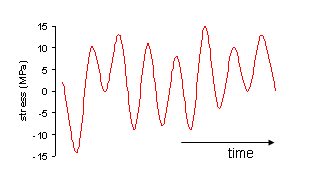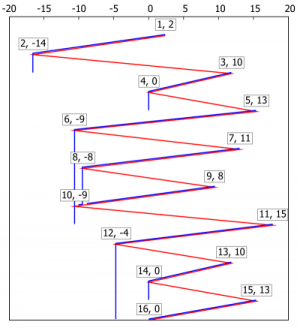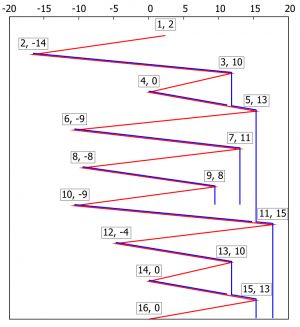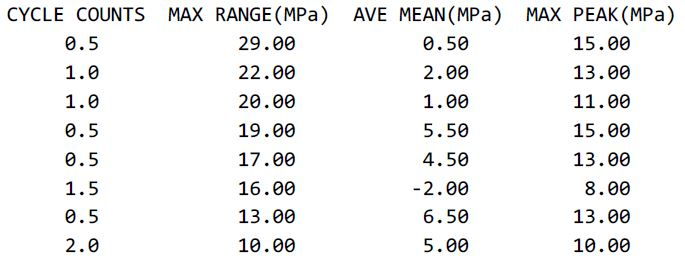# Rainflow CountingFor simple periodic loadings, such as Figure 1, rainflow counting is unnecessary. That sequence clearly has 9.5 cycles of amplitude 10 MPa and a structure’s life can be estimated from a simple application of the relevant S-N curve.Compare this with Figure 2 which cannot be assessed in terms of simply-described stress reversals.## Rainflow Counting Algorithm

The “rainflow” was named from a comparison of this algorithm to the flow of rain falling on a pagoda and running down the edges of the roof. The rainflow counting algorithm consists of the following steps:

1. Reduce the time history to a sequence of (tensile) peaks and (compressive) valleys.
2. Imagine that the time history is a template for a rigid sheet like a pagoda roof.
3. Turn the sheet clockwise 90° (earliest time to the top), and now it looks like a pagoda roof (Figure 3).
4. Each tensile peak is imagined as a source of water that “drips” down the pagoda.
5. Count the number of half-cycles by looking for terminations in the flow occurring when either:
• case (a) It reaches the end of the time history;
• case (b) It merges with a flow that started at an earlier tensile peak; or
• case (c) It flows when an opposite tensile peak has greater or equal magnitude.
6. Repeat step 5 for compressive valleys.
7. Assign a stress range Δσ = σmax – σmin to each half-cycle equal to the stress difference between its start and termination.
8. Pair up half-cycles of identical magnitude but opposite sense to count the number of complete cycles. Unmatched half-cycles are residual half-cycles.Figure 3:  Pagoda roof

A given half cycle may contain smaller half cycles.  As a general rule, large stress cycles must not be fragmented into smaller ones as this will lead to underestimation of fatigue damage.  Smaller stress cycles should be treated as temporary interruptions of larger stress reversals.

## Rainflow Counting Example

• The stress history in Figure 2 is reduced to peaks and valleys in Figure 4.  The stress values are 2,-14,10,0,13,-9,11,-8,8,-9,15,-4,10,0,13,0 (MPa).
• The 1st half-cycle starts at tensile peak (1) and terminates opposite a greater tensile stress, peak (3) (case c); the stress range Δσ = 2 – (-14) = 16 MPa.
• The half-cycle starting at peak (9) terminates where it is interrupted by a flow from earlier peak (8) (case b); the stress range Δσ = 8 – (-8) = 16 MPa.
• The half-cycle starting at peak 11 terminates at the end of the time history (case a); the stress range Δσ = 15 – (-4) = 19 MPa.
• Similar half-cycles are calculated for compressive stresses (Figure 5) and the half-cycles are then matched.  A pair of matched half cycles (one tension, one compression) is counted as a full cycle (or 1 cycle); an unmatched half-cycle is counted as a half cycle (or 0.5 cycle).Figure 4:  Rainflow for tensile peaksFigure 5:  Rainflow for compressive valleys

The results are summarized in the following table.  Note that a half cycle is equivalent to 0.5 full cycle.

 Stress Range Δσ (MPa) Full Cycles Half Cycles Total Full Cycles 10 2 0 2 13 0 1 0.5 16 1 1 1.5 17 0 1 0.5 19 0 1 0.5 20 1 0 1 22 1 0 1 29 0 1 0.5

Watch the following video and see how the results in the above table are calculated:

The cycle counting results match those calculated in Fatigue+ software as shown below:For details of Fatigue+ software, click here.

## ASTM E1049-85 (2017) Rainflow Counting ExampleFigure 6: Rainflow Counting Example

The following is an example of Fatigue+ software.  The unit of the stresses is MPa.  The stress history is taken from FIG. 6 of ASTM E1049, Standard Practices for Cycle Counting in Fatigue Analysis, amplified by 10 times.